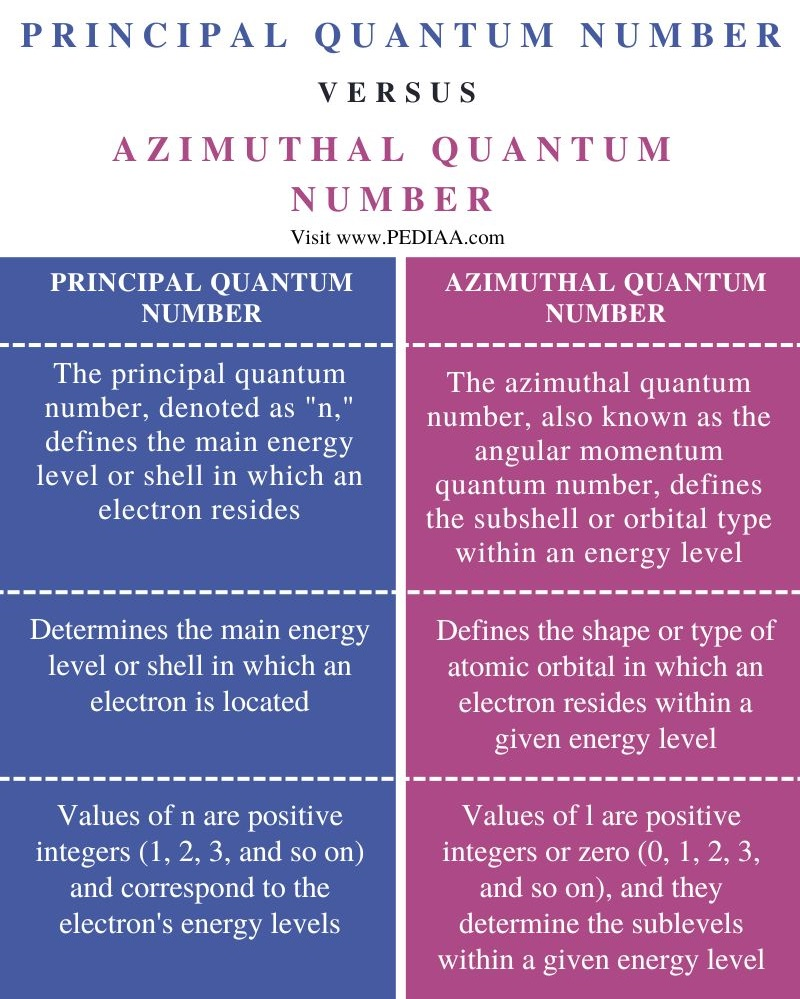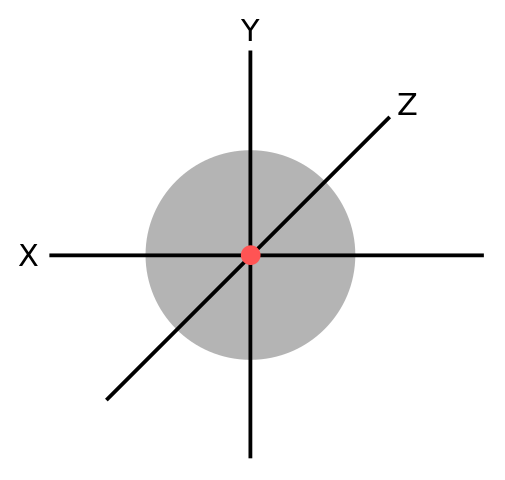# What is the Difference Between Principal and Azimuthal Quantum Number

The main difference between principal and azimuthal quantum number is that the principal quantum number, represented by the symbol ‘n,’ determines the main energy level or shell in which an electron is located, whereas azimuthal quantum number, represented by the symbol ‘l,’ is responsible for defining the shape or type of orbital in which an electron resides.

Principal Quantum Number (n) and Azimuthal Quantum Number (l) are quantum numbers that help to describe the behavior of electrons within atoms according to the principles of quantum mechanics. Both numbers serve a specific role in characterizing an electron’s properties and its location within an atom.

### Key Areas Covered

1. What is the Principal Quantum Number
– Definition, Features
2. What is the Azimuthal Quantum Number
– Definition, Features
3. Similarities Between Principal and Azimuthal Quantum Number
– Outline of Common Features
4. Difference Between Principal and Azimuthal Quantum Number
– Comparison of Key Differences
5. FAQ: Principal and Azimuthal Quantum Number

### Key Terms

Principal Quantum Number, Azimuthal Quantum Number## What is the Principal Quantum Number

The principal quantum number, represented by the symbol “n,” is the most significant quantum number when it comes to understanding the energy levels of electrons in an atom. It defines the main energy level or shell in which an electron is located. It tells us how far an electron is from the nucleus of the atom and provides information about the electron’s energy. The principal quantum number essentially divides the electrons into energy levels or shells within an atom. Each energy level corresponds to a specific value of n. As electrons occupy different energy levels, they have varying average distances from the nucleus and exhibit different energy levels. These energy levels are often referred to as K, L, M, N, and so on, with K being the energy level closest to the nucleus (n = 1), L being the next (n = 2), and so forth.The principal quantum number is intricately linked to the overall structure of an atom. Electrons within the same energy level (same n value) share similar average distances from the nucleus and energy. As n increases, the energy level moves farther from the nucleus, and the electron’s energy increases. Electrons with higher values of n have a greater potential energy.

The principal quantum number also has direct applications in the periodic table. Elements are arranged in the periodic table based on the number of protons in their nucleus, which determines the atomic number. The atomic number, in turn, is associated with the number of electrons in the atom. The principal quantum number helps explain the organization of the periodic table into periods (rows) and groups (columns), as it indicates the main energy levels and electron configuration for each element. The concept of the principal quantum number is fundamental in the field of spectroscopy.

## What is the Azimuthal Quantum Number

The azimuthal quantum number provides insights into the shapes and types of atomic orbitals, which are the regions in space where electrons are most likely to be found. This number plays a vital role in understanding the three-dimensional spatial distribution of electrons in an atom. It is responsible for defining the shape or type of atomic orbital in which an electron resides within a given energy level. It quantifies the angular momentum of the electron’s motion. The values of l determine the specific orbital shapes, and they are integral to the understanding of the spatial distribution of electrons in an atom.

### Electron Sublevels

The azimuthal quantum number categorizes electrons into sublevels, often referred to as subshells, within a given energy level. These sublevels represent different orbital shapes, with each value of l corresponding to a specific shape:

When l =

0 ⇒ designates an s sublevel, characterized by spherical-shaped orbitals.

1 ⇒ represents a p sublevel, where orbitals have a dumbbell or figure-eight shape.

2 ⇒ corresponds to a d sublevel, with more complex, multifaceted orbital shapes.

3 ⇒ designates an f sublevel, with intricate and even more complex orbital shapes.

The azimuthal quantum number is crucial in determining the possible shapes of atomic orbitals within a given energy level.For any given energy level with a principal quantum number (n), the azimuthal quantum number (l) can take on values ranging from 0 to (n-1). This means that the value of n determines the number of possible sublevels or subshells. For instance, in the first energy level (n = 1), there is only one sublevel (l = 0), which is an s sublevel. In the second energy level (n = 2), there are two sublevels (l = 0 and l = 1) corresponding to s and p subshells.

## Similarities Between Principle and Azimuthal Quantum Number

• Both are quantum numbers.
• Both are integral to electron configuration.

## Difference Between Principal and Azimuthal Quantum Number

### Definition

The principal quantum number, denoted as “n,” defines the main energy level or shell in which an electron resides, whereas the azimuthal quantum number, also known as the angular momentum quantum number, defines the subshell or orbital type within an energy level.

### Function

The principal quantum number determines the main energy level or shell in which an electron is located, while the azimuthal quantum number defines the shape or type of atomic orbital in which an electron resides within a given energy level.

### Values

The values of n are positive integers (1, 2, 3, and so on) and correspond to the electron’s energy levels, while the values of l are positive integers or zero (0, 1, 2, 3, and so on), and they determine the sublevels within a given energy level.

## FAQ: Principal and Azimuthal Quantum Number

### What is the relationship between principal quantum number and azimuthal quantum number?

The principal quantum number (n) determines the main energy level or shell, while the azimuthal quantum number (l) defines the subshell or orbital type within that energy level. In other words, the value of “n” dictates the overall energy level, while “l” further specifies the shape and characteristics of the orbitals within that energy level.

### What is the formula of azimuthal quantum number?

The formula for the azimuthal quantum number (l) is not a mathematical equation like a formula with specific variables. Instead, it’s represented as a whole number that can take on values from 0 to (n-1), where “n” is the principal quantum number.

### Can the principal quantum number be 0?

No, the principal quantum number cannot be 0. It always takes positive integer values, typically starting from 1 and increasing in whole numbers (1, 2, 3, and so on), to represent different energy levels or shells in an atom.

## Conclusion

The principal quantum number determines the main energy level or shell in which an electron is located, while the azimuthal quantum number defines the shape or type of atomic orbital in which an electron resides within a given energy level. Thus, this is the main difference between principal and azimuthal quantum number.

##### Reference:

1. “Principal Quantum Number.” Wikipedia. Wikipedia Foundation.
2. “Azimuthal Quantum Number.” Byju’s.

##### Image Courtesy:

1. “Orbitals-chart-by-quantum-number” By MikeRun – Own work (CC BY-SA 4.0) via Commons Wikimedia
2. “Principal quantum number – large” By Richard Parsons (CC BY-SA 3.0) via Commons Wikimedia### About the Author: Hasini A

Hasini is a graduate of Applied Science with a strong background in forestry, environmental science, chemistry, and management science. She is an amateur photographer with a keen interest in exploring the wonders of nature and science.